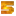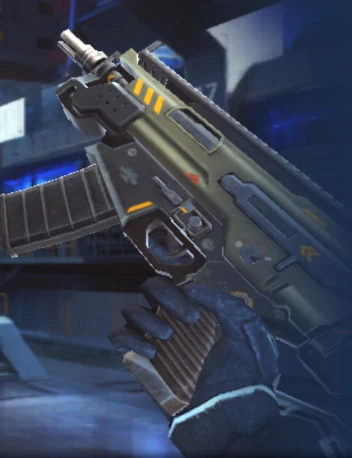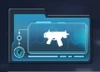The SIX-MG is a submachine gun in Modern Combat 5: Blackout.

## Modern Combat 5: Blackout

 DB‑HG SIX‑MG XSSG Micro COV CO‑MA 11 Severance H.C.‑BDOG ST‑2xel A.E.S.T.‑X84

{{#infoboxbuilder: | 0:Theme = MC5 default | 0:CustomModule = | 0:MainImageCaption = | 0:ToggleContentLongerThan = 1500

| 1:Type = Title

```| 1:Value = SIX-MG
```

| 2:Type = MainImage

```| 2:Value =```

| 3:Type = Line

```| 3:Label = Type
| 3:Value = Submachine Gun
```

| 4:Type = Line

```| 4:Label = Likely based on
| 4:Value = SIG MPX-K
```

| 5:Type = Line

```| 5:Label = Users
| 5:Value =
```

| 6:Type = Line

```| 6:Label = Manufacturer
| 6:Value =
```

| 7:Value =

Statistics

| 8:Type = Line

```| 8:Label = Damage
| 8:Value =
```

| 9:Type = Line

```| 9:Label = Radius
| 9:Value =
```

| 10:Type = Line

```| 10:Label = Rate of fire
| 10:Value =
```

| 11:Type = Line

```| 11:Label = Range
| 11:Value =
```

| 12:Type = Line

```| 12:Label = Accuracy
| 12:Value =
```

| 13:Type = Line

```| 13:Label = Handling
| 13:Value =
```

| 14:Type = Line

```| 14:Label = Mobility
| 14:Value =
```

| 15:Type = Line

```| 15:Label = Magazine size
| 15:Value =
```

| 16:Type = Line

```| 16:Label = Starting ammo
| 16:Value =
```

| 17:Type = Line

```| 17:Label = Max. ammo
| 17:Value =
```

| 18:Type = Line

```| 18:Label = Ammo received via pickup
| 18:Value =
```

| 19:Type = Line

```| 19:Label = Reloading
| 19:Value =
```

| 20:Type = Line

```| 20:Label = Fire mode
| 20:Value =
```

| 21:Type = Line

```| 21:Label = Effect
| 21:Value =

```

| 22:Type = Line

```| 22:Label = Max Capacity
| 22:Value =
```

| 23:Type = Line

```| 23:Label = Stealth
| 23:Value =
```

| 24:Type = Line

```| 24:Label = Countered by
| 24:Value =
```

| 25:Value =

Availability

| 26:Type = Line

```| 26:Label = Class
| 26:Value = Kommander
```

| 27:Type = Line

```| 27:Label = Tier
| 27:Value = T2
```

| 28:Type = Line

```| 28:Label = Unlocked
| 28:Value =
```

| 29:Type = Line

```| 29:Label = Cost
| 29:Value =
```

| 30:Type = Image

```| 30:Value =```

| 31:Type = Footer

```| 31:Value =
```

}}

The SIX-MG is the T2 light tactical gun of the Kommander class. It is a submachine gun sporting an extremely high rate of fire, but very low damage as well as significant damage drop-off, with higher-tier weapons in its class generally being preferred over it.

 + Extremely high rate of fire Fast reload − Very low damage Low effective range Cannot equip scopes with 4X zoom